# 16.1 The Path-Space Measurement Equation

measurement equation（测量方程）描述了一种由积分一系列带有辐照度的光线得到的抽象的度量。例如在最简单的，最简化的成像模型中计算图像中的像素 $j$ 的情况下，我们可以把该像素的值写成在图像平面上积分的如下形式：

\begin{aligned}I_{j} &=\int_{A_{\text {film }}} \int_{\mathrm{S}^{2}} W_{\mathrm{e}}^{(j)}\left(\mathrm{p}_{\text {film }}, \omega\right) L_{\mathrm{i}}\left(\mathrm{p}_{\text {film }}, \omega\right)|\cos \theta| \mathrm{d} \omega \mathrm{d} A\left(\mathrm{p}_{\text {film }}\right) \\&=\int_{A_{\text {film }}} \int_{A} W_{\mathrm{e}}^{(j)}\left(\mathrm{p}_{0} \rightarrow \mathrm{p}_{1}\right) L\left(\mathrm{p}_{1} \rightarrow \mathrm{p}_{0}\right) G\left(\mathrm{p}_{0} \leftrightarrow \mathrm{p}_{1}\right) \mathrm{d} A\left(\mathrm{p}_{1}\right) \mathrm{d} A\left(\mathrm{p}_{0}\right)\end{aligned}

$W_{\mathrm{e}}^{(j)}\left(\mathrm{p}_{0} \rightarrow \mathrm{p}_{1}\right)=f_{j}\left(\mathrm{p}_{0}\right) \delta\left(t\left(\mathrm{p}_{0}, \omega_{\text {camera }}\left(\mathrm{p}_{1}\right)\right)-\mathrm{p}_{1}\right)$

\begin{aligned}I_{j} &=\int_{A_{\mathrm{film}}} &\int_{A} W_{\mathrm{e}}^{(j)}\left(\mathrm{p}_{0} \rightarrow \mathrm{p}_{1}\right) L\left(\mathrm{p}_{1} \rightarrow \mathrm{p}_{0}\right) G\left(\mathrm{p}_{0} \leftrightarrow \mathrm{p}_{1}\right) \mathrm{d} A\left(\mathrm{p}_{1}\right) \mathrm{d} A\left(\mathrm{p}_{0}\right) \\&=\sum_{i} &\int_{A} \int_{A} W_{\mathrm{e}}^{(j)}\left(\mathrm{p}_{0} \rightarrow \mathrm{p}_{1}\right) P\left(\overline{\mathrm{p}}_{i}\right) G\left(\mathrm{p}_{0} \leftrightarrow \mathrm{p}_{1}\right) \mathrm{d} A\left(\mathrm{p}_{1}\right) \mathrm{d} A\left(\mathrm{p}_{0}\right) \\&=\sum_{i} &\underbrace{\int_{A} \cdots \int_{A}}_{i+1 \text { times }} W_{\mathrm{e}}^{(j)}\left(\mathrm{p}_{0} \rightarrow \mathrm{p}_{1}\right) T\left(\overline{\mathrm{p}}_{i}\right) L_{\mathrm{e}}\left(\mathrm{p}_{\mathrm{i}+1} \rightarrow \mathrm{p}_{\mathrm{i}}\right) G\left(\mathrm{p}_{0} \leftrightarrow \mathrm{p}_{1}\right) \\&&\mathrm{d} A\left(\mathrm{p}_{\mathrm{i}+1}\right) \cdots \mathrm{d} A\left(\mathrm{p}_{0}\right) \end{aligned}

## 16.1.1 采样相机光线

1. 计算光线和摄像机朝向的夹角，检查光线是否和摄像机朝向一致
2. 计算光栅位置，并按需写入参数指定的地址
3. 对于采样区域外的位置，返回零值
4. 计算重要性的函数值

PerspectiveCamera 类在定义上为了防止出现物理中的小孔成像的暗角问题，在 PDF 设计上加入了光线方向的影响。对于面积为 $A$ 的成像平面，其 PDF 定义为：

$p(\omega) = {d^2 \over A\cos^3\theta} ,\ \mathrm{if \ \omega \ is \ within \ frustum, \ else \ 0}$

$p(\omega) = {d^2 \over A\pi r^2\cos^4\theta} ,\ \mathrm{if \ \omega \ is \ within \ frustum, \ else \ 0}$

## 16.1.2 采样光源光线

### 方向光## 16.1.3 非对称散射

$T\left(\overline{\mathrm{p}}_{n}\right)=\prod_{i=1}^{n-1} f\left(\mathrm{p}_{i+1} \rightarrow \mathrm{p}_{i} \rightarrow \mathrm{p}_{i-1}\right) G\left(\mathrm{p}_{i+1} \leftrightarrow \mathrm{p}_{i}\right)$

### 折射带来的非对称性

$L_i = \frac{\eta_i^2}{\eta_t^2}L_t$

$f^{*}\left(\mathrm{p}, \omega_{\mathrm{o}}, \omega_{\mathrm{i}}\right)=f\left(\mathrm{p}, \omega_{\mathrm{i}}, \omega_{\mathrm{o}}\right)=\frac{\eta_{\mathrm{t}}^{2}}{\eta_{\mathrm{i}}^{2}} f\left(\mathrm{p}, \omega_{\mathrm{o}}, \omega_{\mathrm{i}}\right)$

### 着色法线带来的非对称性

$L_{\mathrm{o}}\left(\mathrm{p}, \omega_{\mathrm{o}}\right)=L_{\mathrm{e}}\left(\mathrm{p}, \omega_{\mathrm{o}}\right)+\int_{\mathrm{S}^{2}} f\left(\mathrm{p}, \omega_{\mathrm{o}}, \omega_{\mathrm{i}}\right) L_{\mathrm{i}}\left(\mathrm{p}, \omega_{\mathrm{i}}\right)\left|\mathbf{n}_{\mathrm{g}} \cdot \omega_{\mathrm{i}}\right| \mathrm{d} \omega_{\mathrm{i}}$

$f_{shade}(p, \omega_o, \omega_i) = {|\mathbf{n}_s \cdot \omega_i| \over |\mathbf{n}_g \cdot \omega_i|} f(p, \omega_o, \omega_i)\\ f_{shade}^*(p, \omega_o, \omega_i) = {|\mathbf{n}_s \cdot \omega_o| \over |\mathbf{n}_g \cdot \omega_o|} f^*(p, \omega_o, \omega_i)$

$f^*(p, \omega_o, \omega_i) = {|\mathbf{n}_s \cdot \omega_o||\mathbf{n}_g \cdot \omega_i| \over |\mathbf{n}_g \cdot \omega_o||\mathbf{n}_s \cdot \omega_i|}f(p, \omega_o, \omega_i), \mathrm{while \ transporting \ importance}$

# 16.2 Stochastic Progressive Photon Mapping (SPPM)

## 16.2.1 理论基础$E\left[\frac{1}{N} \sum_{j=1}^{N} \beta_{j} W_{\mathrm{e}}\left(\mathrm{p}_{\mathrm{j}}, \omega_{\mathrm{j}}\right)\right]=\int_{A} \int_{\mathrm{S}^{2}} W_{\mathrm{e}}(\mathrm{p}, \omega) L_{\mathrm{i}}(\mathrm{p}, \omega)|\cos \theta| \mathrm{d} A \mathrm{~d} \omega$

$E\left[\frac{1}{N} \sum_{i=1}^{N} W_{\mathrm{e}}\left(\mathrm{p}_{\mathrm{i}, 0} \rightarrow \mathrm{p}_{\mathrm{i}, 1}\right)\left\{\frac{L\left(\mathrm{p}_{\mathrm{i}, 1} \rightarrow \mathrm{p}_{\mathrm{i}, 0}\right) G\left(\mathrm{p}_{\mathrm{i}, 0} \leftrightarrow \mathrm{p}_{\mathrm{i}, 1}\right)}{p\left(\mathrm{p}_{\mathrm{i}, 0}\right) p\left(\mathrm{p}_{\mathrm{i}, 1}\right)}\right\}\right]$

$\beta_{i, j}=\frac{L_{\mathrm{e}}\left(\mathrm{p}_{\mathrm{i}, \mathrm{n}_{\mathrm{i}}} \rightarrow \mathrm{p}_{\mathrm{i}, \mathrm{n}_{\mathrm{i}}-1}\right)}{p\left(\mathrm{p}_{\mathrm{i}, \mathrm{n}_{\mathrm{i}}}\right)} \prod_{j=1}^{n_{i}-1} \frac{1}{1-q_{i, j}} \frac{f\left(\mathrm{p}_{\mathrm{i}, \mathrm{j}+1} \rightarrow \mathrm{p}_{\mathrm{i}, \mathrm{j}} \rightarrow \mathrm{p}_{\mathrm{i}, \mathrm{j}-1}\right) G\left(\mathrm{p}_{\mathrm{i}, \mathrm{j}+1} \leftrightarrow \mathrm{p}_{\mathrm{i}, \mathrm{j}}\right)}{p\left(\mathrm{p}_{\mathrm{i}, \mathrm{j}}\right)}$

## 16.2.2 光子映射（Photon Mapping）

\begin{aligned}\int_{\mathrm{S}^{2}} & L_{\mathrm{i}}\left(\mathrm{p}, \omega_{\mathrm{i}}\right) f\left(\mathrm{p}, \omega_{\mathrm{o}}, \omega_{\mathrm{i}}\right)\left|\cos \theta_{\mathrm{i}}\right| \mathrm{d} \omega_{\mathrm{i}} \\&=\int_{A} \int_{\mathrm{S}^{2}} \delta\left(\mathrm{p}-\mathrm{p}^{\prime}\right) L_{\mathrm{i}}\left(\mathrm{p}^{\prime}, \omega_{\mathrm{i}}\right) f\left(\mathrm{p}^{\prime}, \omega_{0}, \omega_{\mathrm{i}}\right)\left|\cos \theta_{\mathrm{i}}\right| \mathrm{d} \omega_{\mathrm{i}} \mathrm{d} A\left(\mathrm{p}^{\prime}\right)\end{aligned}

$W_e(p', \omega) = \delta(p' - p)f(p, \omega_o, \omega)$$\hat{p}(x) = {1 \over Nh}\sum_{i=1}^N k({x - x_i \over h})$$\hat{p}(x) = {1 \over Nd_N(x)}\sum_{i=1}^N k({x - x_i \over d_N(x)})$

$\hat{p}(x) = {1 \over N(d_N(x))^d}\sum_{i=1}^N k({x - x_i \over d_N(x)})$

$L_{\mathrm{o}}\left(\mathrm{p}, \omega_{\mathrm{o}}\right) \approx \frac{1}{N_{\mathrm{p}} d_{N}(\mathrm{p})^{2}} \sum_{j}^{N_{\mathrm{p}}} k\left(\frac{\mathrm{p}-\mathrm{p}_{j}}{d_{N}(\mathrm{p})}\right) \beta_{j} f\left(\mathrm{p}, \omega_{\mathrm{o}}, \omega_{j}\right)$

### Progressive Photon Mapping

PPM 算法重构了光子映射的流程以解决这一问题：首先进行的相机 pass 向场景中追踪相机射线，在每个像素中储存所有非镜面的表面信息，这些信息被称为可视点（visible points）。接着的第二个 pass 则负责从光源跟踪光子，在每个光子与表面的作用位置向周围的可视点贡献照明。观察展开后的 LTE ：

\begin{aligned}L\left(\mathrm{p}, \omega_{\mathrm{o}}\right) &=L_{\mathrm{e}}\left(\mathrm{p}, \omega_{\mathrm{o}}\right)+\int_{\mathrm{S}^{2}} f\left(\mathrm{p}, \omega_{\mathrm{o}}, \omega_{\mathrm{i}}\right) L\left(\mathrm{p}, \omega_{\mathrm{i}}\right)\left|\cos \theta_{\mathrm{i}}\right| \mathrm{d} \omega_{\mathrm{i}} \\&=L_{\mathrm{e}}\left(\mathrm{p}, \omega_{\mathrm{o}}\right)+\int_{\mathrm{S}^{2}} f\left(\mathrm{p}, \omega_{\mathrm{o}}, \omega_{\mathrm{i}}\right) L_{\mathrm{d}}\left(\mathrm{p}, \omega_{\mathrm{i}}\right)\left|\cos \theta_{\mathrm{i}}\right| \mathrm{d} \omega_{\mathrm{i}} \\&+\int_{\mathrm{S}^{2}} f\left(\mathrm{p}, \omega_{\mathrm{o}}, \omega_{\mathrm{i}}\right) L_{\mathrm{i}}\left(\mathrm{p}, \omega_{\mathrm{i}}\right)\left|\cos \theta_{\mathrm{i}}\right| \mathrm{d} \omega_{\mathrm{i}}\end{aligned}

### Stochastic Progressive Photon Mapping

SPPM 在 PPM 的基础上作出了改进，使得光子映射算法不必受到相机光线采样的内存性能影响。和 PPM 一样，它会生成一系列的可视点记录，但不同的是每次生成的记录采样率都比较低，接着发射光子，记录数值，接着丢弃所有的现有采样点，重复这一步骤。

SPPM 对光子近似函数进行了两个修改，首先，它使用了一个固定的圆盘状核函数：

$L_o(p,\omega_o) \approx {1 \over N_p\pi r^2}\sum_j^{N_p}\beta_j f(p,\omega_o,\omega_j)$

\begin{aligned}N_{i+1} &=N_{i}+\gamma M_{i} \\r_{i+1} &=r_{i} \sqrt{\frac{N_{i+1}}{N_{i}+M_{i}}} \\\tau_{i+1} &=\left(\tau_{i}+\Phi_{i}\right) \frac{r_{i+1}^{2}}{r_{i}^{2}}\end{aligned}

## 16.2.3 SPPMIntegrator

SPPMIntegrator 不是一个 SamplerIntegrator ，因此它实现了自己的 Render() 方法。它会在初始化一系列相关变量后运行多次 SPPM 迭代。

SPPM 的像素数据储存在结构体 SPPMPixel 中，其中除了估计结果外还包含了一系列运行时变量和可视点的信息：

SamplerIntegrator 相似，SPPMIntegrator 也将图像分割为 16 * 16 像素的子块并行地渲染。在完成一系列采样器设置、内存管理初始化后，会进入迭代环节，并按顺序完成以下环节：

1. 生成 SPPM 可视点
2. 跟踪光子并累积贡献度
3. 更新像素值，并周期性地将中间结果保存下来

## 16.2.5 构建可视点网格结构1. 顺序遍历所有有效可视点，生成最小全覆盖网格的 AABB ，并记录最大搜索半径
2. 生成边长约为最大搜索半径的方形网格
3. 并行遍历所有有效可视点，将每个可视点按影响范围写入网格

## 16.2.6 累积光子贡献

$\beta = {|\cos\omega_0|L_e(p_0,\omega_0) \over p(\mathrm{light})p(p_0,\omega_0)}$

$L = L_d + {\tau \over N_p\pi r^2}$

# 16.3 Bidirectional Path TracingBDPT 基于从摄像机和光源两个位置生成两个子路径，接着连接路径的端点从而生成一个完整的路径。从表面上看，这一过程和光子映射中的两个步骤相似度极高，但这两种方法实际上在构建和处理光路上有着巨大的差别。

1. 重用子路径：给定一条光路 $q_0, q_1, \cdots, q_{s-1}, p_{t-1}, \cdots, p_1, p_0$ ，其中任意的子序列均可能构成新的有效光路。
2. 抛弃可以使用直接光照或直接重要性传输快速求解的路径第一个节点带来的光照
3. 使用不同的权重对使用不同策略生成的光路进行加权，对于同一条包含 $n$ 次散射的光路而言，一共有 $n+3$ 种不同的链接方法，其中部分策略可能比别的策略更加有效，这意味着 MIS 也可以被引入路径的构建中SPPMIntegrator 一样，BDPTIntegrator 也直接继承自 Integrator 类。所有子路径生成均在 BDPTIntegrator::Render() 中经由一个嵌套循环完成，且过程也十分相似：

1. 细分图像为 $16 \times 16$ 的子块
2. 对每个子块生成内存管理模块和采样器
3. 对于每个像素，重复采样直到 Sampler::StartNextSample() 返回 false

## 16.3.1 Vertex 抽象层

PBRT 中总共有 4 种路径顶点类型：enum class VertexType { Camera, Light, Surface, Medium };

• 统一 BSDF 和 phase function 的传输函数 f
• 判断表面类型、是否可连接的一系列函数 IsOnSurface, IsLight, IsConnectable...
• 根据给定前后节点计算该次采样 PDF 的 Pdf

## 16.3.2 生成子路径

RandomWalk 函数中，pdfFwd, pdfRev 两个局部变量在每个光线追踪的迭代之间更新，它们满足以下情况：

1. 在迭代开始时，pdfFwd 记录了当前的采样射线 ray.d 在立体角上的概率
2. pdfFwd 会在创建 Vertex 时被转换为单位平面上的概率储存在当前 Vertex::pdfFwd
3. 在迭代结束时，pdfRev 记录了在已知新的出射方向时，将旧射线端点交换后得到的反向射线的立体角概率
4. pdfRev 会被转换并写入前一个节点的 Vertex::pdfRev

## 16.3.3 连接子路径

ConnectBDPT 函数的作用是，输入两段子路径和 s, t 两个参数表示两侧分别选取的节点数，返回对应连接策略的贡献值。特别地，当 t = 1 即只使用一个摄像机节点时，对应的胶片位置会使用 pRaster 返回。

• 对于非法连接（连接灯光路径和摄像机路径中的灯光）直接返回零值

• t == 1 时：忽略灯光路径信息，而使用和 PT 相同的直接光照估计方法计算

• s == 0 时：将相机路径视为一个完整路径处理，返回相机路径最后一点的出射照度对整个路径的贡献

• s == 1 时：在返回贡献的同时返回像素位置

• 在其它情况下，在测试连接性后，返回贡献度，其中使用 G(scene, sampler, qs, pt) 函数返回两点之间的几何项，公式如下：

$\begin{array}{r}\hat{P}\left(\overline{\mathrm{q}}_{s} \overline{\mathrm{p}}_{t}\right)=L_{\mathrm{e}} \hat{T}\left(\overline{\mathrm{q}}_{s}\right)\left[\hat{f}\left(\mathrm{q}_{s-2} \rightarrow \mathrm{q}_{s-1} \rightarrow \mathrm{p}_{t-1}\right) \hat{G}\left(\mathrm{q}_{s-1} \leftrightarrow \mathrm{p}_{\mathrm{t}-1}\right)\right. \\\left.\hat{f}\left(\mathrm{q}_{s-1} \rightarrow \mathrm{p}_{t-1} \rightarrow \mathrm{p}_{t-2}\right)\right] \hat{T}\left(\overline{\mathrm{p}}_{t}\right) W_{\mathrm{e}}\end{array}$

## 16.3.4 MIS

MIS 在 BDPT 中发挥了重要的作用，通过赋予不同的连接方式以不同的权重，这一方法可以大幅降低结果的方差。为了实现在路径上的 MIS ，我们需要一种方法以获知每一种路径各自的概率。这一步发生在 ConnectBDPT 函数的最后，它调用 MISWeight ，利用路径信息，特别是之前缓存的 pdfFwd, pdfRev ，以生成对应的概率。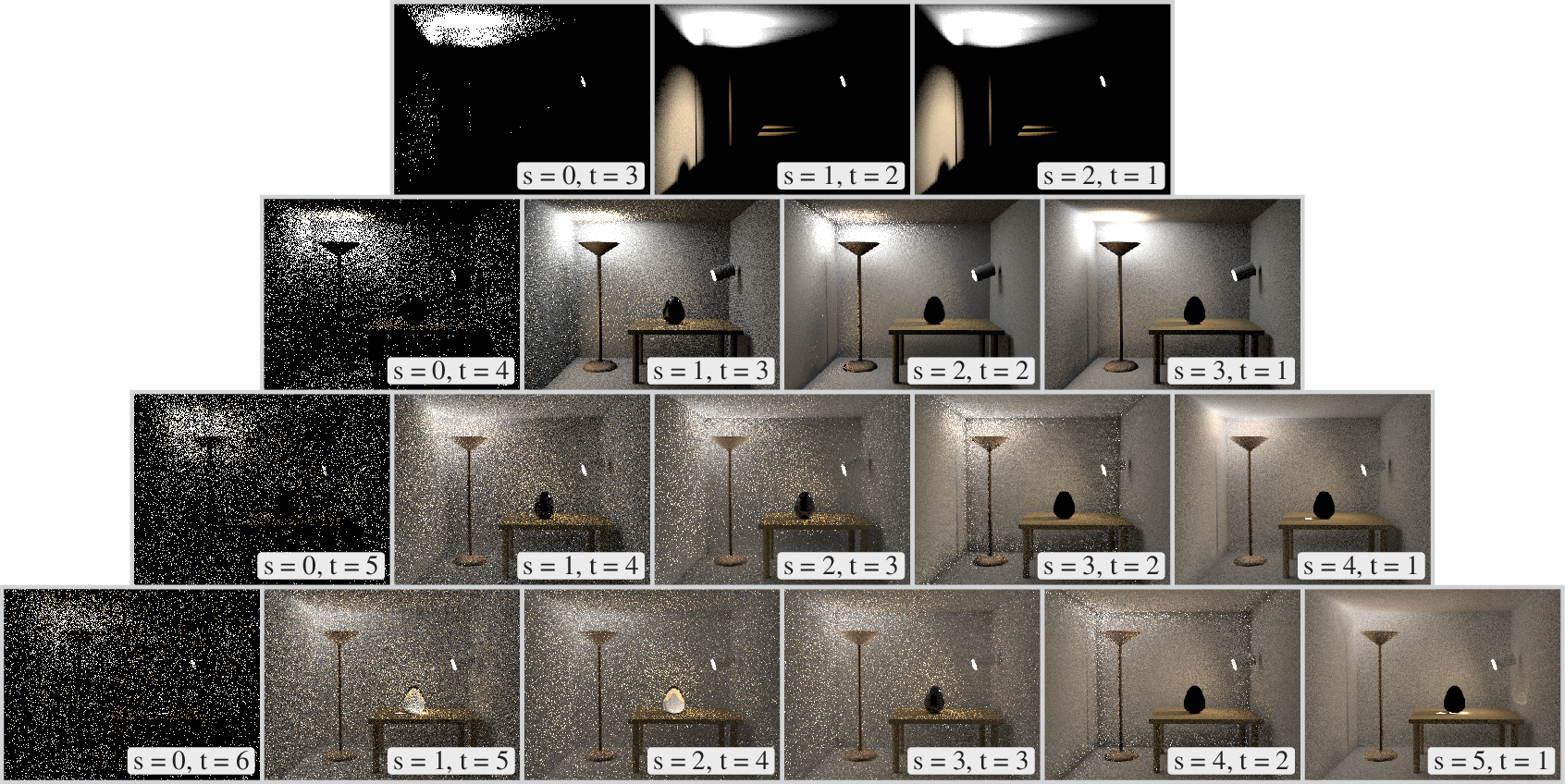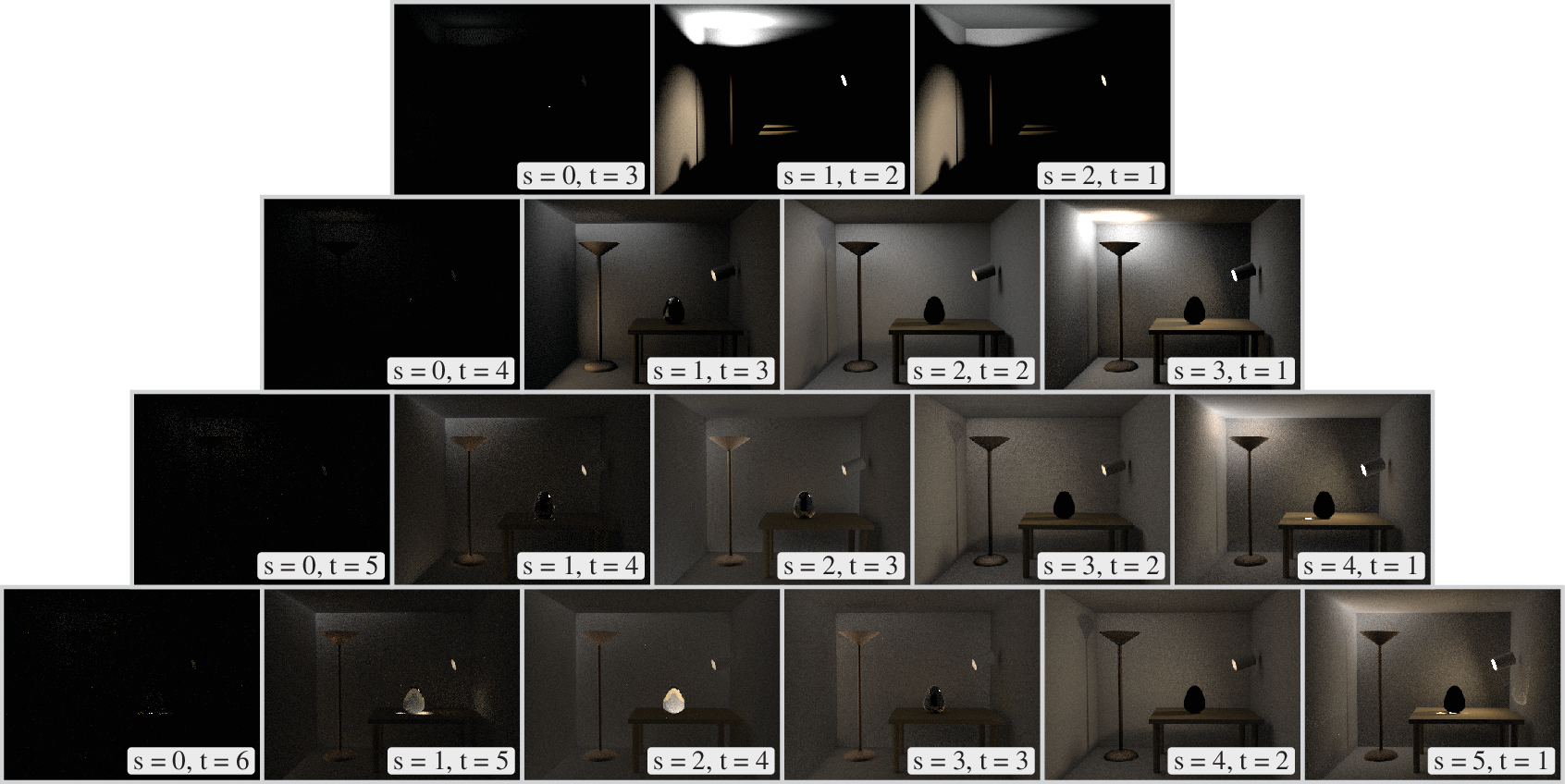$\overline{\mathrm{x}}=\left(\mathrm{x}_{0}, \ldots, \mathrm{x}_{\mathrm{n}-1}\right)=\left(\mathrm{q}_{0}, \ldots, \mathrm{q}_{s-1}, \mathrm{p}_{t-1}, \ldots, \mathrm{p}_{0}\right)$

$p_{s}(\overline{\mathrm{x}})=p^{\rightarrow}\left(\mathrm{x}_{0}\right) \cdots p^{\rightarrow}\left(\mathrm{x}_{\mathrm{S}-1}\right) \cdot p^{\leftarrow}\left(\mathrm{x}_{\mathrm{S}}\right) \cdots p^{\leftarrow}\left(\mathrm{x}_{\mathrm{n}-1}\right)$

$p_{i}(\overline{\mathrm{x}})=p^{\rightarrow}\left(\mathrm{x}_{0}\right) \cdots p^{\rightarrow}\left(\mathrm{x}_{i-1}\right) \cdot p^{\leftarrow}\left(\mathrm{x}_{i}\right) \cdots p^{\leftarrow}\left(\mathrm{x}_{\mathrm{n}-1}\right), \ 0 \leq i \leq n$

$w_{s}(\overline{\mathrm{x}})=\frac{p_{s}(\overline{\mathrm{x}})}{\sum_{i} p_{i}(\overline{\mathrm{x}})}$

1. 路径密度函数很容易溢出浮点数的精度表示，它的精度要求会随着路径长度和场景复杂度指数增长
2. 原始的 MIS 算法实现会花费相对最大路径长度而言 $O(n^4)$ 的时间复杂度（计算单一概率、总概率和枚举两个路径参数分别会贡献 $O(n)$ 的复杂度）

$w_{s}(\overline{\mathrm{x}})=\frac{1}{\sum_{i} \frac{p_{i}(\overline{\mathrm{x}})}{p_{s}(\overline{\mathrm{x}})}}=\left(\sum_{i=0}^{s-1} \frac{p_{i}(\overline{\mathrm{x}})}{p_{s}(\overline{\mathrm{x}})}+1+\sum_{i=s+1}^{n} \frac{p_{i}(\overline{\mathrm{x}})}{p_{s}(\overline{\mathrm{x}})}\right)^{-1}$

$r_{i}(\overline{\mathrm{x}})=\frac{p_{i}(\overline{\mathrm{x}})}{p_{s}(\overline{\mathrm{x}})}$

\begin{aligned}&r_{i}(\overline{\mathrm{x}})=\frac{p_{i}(\overline{\mathrm{x}})}{p_{i+1}(\overline{\mathrm{x}})} \frac{p_{i+1}(\overline{\mathrm{x}})}{p_{s}(\overline{\mathrm{x}})}=\frac{p_{i}(\overline{\mathrm{x}})}{p_{i+1}(\overline{\mathrm{x}})} r_{i+1}(\overline{\mathrm{x}}) \quad(is)\end{aligned}

\begin{aligned}\frac{p_{i}(\overline{\mathrm{x}})}{p_{i+1}(\overline{\mathrm{x}})} &=\frac{p^{\rightarrow}\left(\mathrm{x}_{0}\right) \cdots p \rightarrow\left(\mathrm{x}_{\mathrm{i}-1}\right) \cdot p^{\leftarrow}\left(\mathrm{x}_{\mathrm{i}}\right) \cdot p^{\leftarrow}\left(\mathrm{x}_{\mathrm{i}+1}\right) \cdots p^{\leftarrow}\left(\mathrm{x}_{\mathrm{n}-1}\right)}{p \rightarrow\left(\mathrm{x}_{0}\right) \cdots p \rightarrow\left(\mathrm{x}_{\mathrm{i}-1}\right) \cdot p \rightarrow\left(\mathrm{x}_{\mathrm{i}}\right) \cdot p^{\leftarrow}\left(\mathrm{x}_{\mathrm{i}+1}\right) \cdots p^{\leftarrow}\left(\mathrm{x}_{\mathrm{n}-1}\right)}=\frac{p^{\leftarrow}\left(\mathrm{x}_{\mathrm{i}}\right)}{p^{\rightarrow}\left(\mathrm{x}_{\mathrm{i}}\right)} \\\frac{p_{i}(\overline{\mathrm{x}})}{p_{\mathrm{i}-1}(\overline{\mathrm{x}})} &=\frac{p^{\rightarrow}\left(\mathrm{x}_{0}\right) \cdots p \rightarrow\left(\mathrm{x}_{\mathrm{i}-2}\right) \cdot p^{\rightarrow}\left(\mathrm{x}_{\mathrm{i}-1}\right) \cdot p^{\leftarrow}\left(\mathrm{x}_{\mathrm{i}}\right) \cdots p^{\leftarrow}\left(\mathrm{x}_{\mathrm{n}-1}\right)}{p \rightarrow\left(\mathrm{x}_{0}\right) \cdots p \rightarrow\left(\mathrm{x}_{\mathrm{i}-2}\right) \cdot p^{\leftarrow}\left(\mathrm{x}_{\mathrm{i}-1}\right) \cdot p^{\leftarrow}\left(\mathrm{x}_{\mathrm{i}}\right) \cdots p^{\leftarrow}\left(\mathrm{x}_{\mathrm{n}-1}\right)}=\frac{p^{\rightarrow}\left(\mathrm{x}_{\mathrm{i}-1}\right)}{p^{\leftarrow}\left(\mathrm{x}_{\mathrm{i}-1}\right)}\end{aligned}

$r_{i}(\overline{\mathrm{x}})= \begin{dcases}1, & \text { if } i=s \\ \frac{p^{\leftarrow}\left(\mathrm{x}_{\mathrm{i}}\right)}{p \rightarrow\left(\mathrm{x}_{\mathrm{i}}\right)} r_{i+1}(\overline{\mathrm{x}}), & \text { if } is\end{dcases}$

## 16.3.5 背景光源和 BDPT

1. 将路径后的第一个端点的 pdfFwd 使用背景光在场景包围球上的面积概率替换
2. 重新计算光源端点的 pdfFwd 为所有背景光源的 pdf 之和

# 16.4 Metropolis Light Transport

1997 年，Veach 和 Guibas 提出了一种非常规的渲染方法，他们称之为 Metropolis Light Transport (MLT)，这种方法将 Metropolis-Hastings 采样算法应用于路径空间的采样之中，使得采样的样本在统计学上具有相关性。MLT 会在场景中生成一系列携带光照的路径，每个路径均由上一个路径衍生而来，并在统计上和各个路径的贡献度具有相同的概率。这种算法拥有极高的灵活性，由于其对演变方法的限制较少，它可以使用一些高度专一化的演变方法以采样对于传统 MC 方法而言难以采样的场景。

## 16.4.1 Primary Sample Space MLT（PSSMLT）

2002 年，Kelemen 等人提出了另一种名为 Primary Sample Space MLT (PSSMLT) 的同样基于 Metropolis-Hastings 采样算法的渲染技术。和原始的 MLT 不同，PSSMLT 并不直接使用路径空间，而是通过附加在 PT 或 BDPT 算法上间接地采样光线。这一做法主要的优势在于 PSSMLT 可以在欧氏空间中使用对称的转移策略，进而更容易被实现，但缺点在于这种算法缺乏对以构建光路的细节信息，从而难以构建和原始 MLT 相似的那种复杂的转移策略。PSSMLT 搜索 PSS 的主要转移策略有两种：

1. 直接替代所有 $X$ 的分量为新的随机生成的数值（大步长）
2. 在各个采样值 $X_i$ 上添加微小的扰动，一般是从以当前位置为中心的正态分布采样（小步长）

## 16.4.2 Multiplexed MLT（MMLT）

2014 年 Hachisuka 等人提出了一种 PSSMLT 的改进算法 Multiplexed Metropolis Light Transport (MMLT) 以解决上述低权重光路的评估问题，MMLT 没有改变每次迭代外的 Metropolis-Hastings 算法过程，而是在 BDPT 的内部增加了细微但有效的改动。和原始 BDPT 中尝试创建所有的路径不同，MMLT 增加了一个随机变量用于选择需要连接的光路，并将该唯一光路的贡献除以其概率并返回。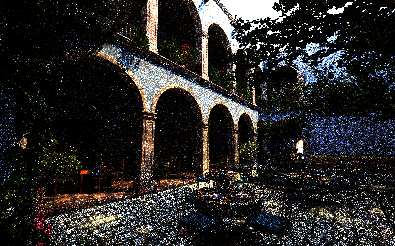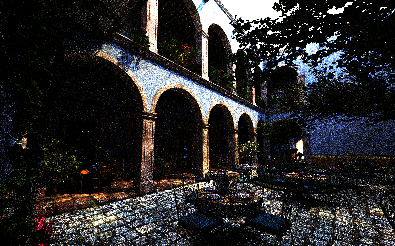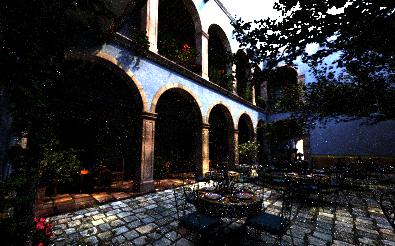PT BDPT MMLT
Modern House 98.0% 97.6% 51.9%
San Miguel 95.9% 97.0% 62.0%

## 16.4.3 渲染中的应用

Metropolis 采样会从给定的标量函数中生成样本，为了将它应用于渲染中，需要解决两个问题：

1. 我们需要对每个像素计算不同的积分以生成整个图片
2. 我们需要处理的函数 $L$ 是一个在光谱上有多个维度的函数而非标量函数

$I_j= \int_\Omega h_j(X)L(X)\mathrm{d}\Omega$

$I_{j} \approx \frac{1}{N} \sum_{i=1}^{N} \frac{h_{j}\left(X_{i}\right) L\left(X_{i}\right)}{p\left(X_{i}\right)}$

$X_i \sim p(X) = {C(X) \over \int_\Omega C(X)\mathrm{d}\Omega}$

$\frac{b}{N} \frac{h_{j}\left(\mathbf{X}_{i}\right) L\left(\mathbf{X}_{i}\right)}{C\left(\mathbf{X}_{i}\right)}$

## 16.4.4 Primary Sample Space Sampler

MLTIntegrator 基于 BDPT 实现了 Metropolis 采样和 MMLT 方法以渲染图片。在介绍该类之前，我们首先需要引入 MLTSampler ，它负责了管理 PSS 的状态变量、变换以及接受 / 拒绝的步骤。

MLTSampler::EnsureReady() 实现了一种懒更新的机制，它会在每次获取值时调用，保证当前调取的值已经被更新。所有的采样值使用 PrimarySample 保存，它除了记录采样值外还额外记录了上一次采样值更新的时间以及一份额外的备份值和备份更新时间，当一个样本被拒绝时，MLTSampler::Reject() 会被调用以恢复所有当前轮次生成的备份值。。特别地，由于正态分布采样有可能采样到超出 $[0,1)$ 范围的数值，在最后还需要将采样值 warp 回规定的范围内：Xi.value -= std::floor(Xi.value)

## 16.4.5 MLT Integrator

### MLTIntegrator::L()

PBRT 的实现使用了三个 MLTSampler 中的样本流，前两个流用于相机和灯光路径采样，而最后一个则用于连接路径中可能出现的采样。

### MLTIntegrator::Render()

1. 首先会生成一系列的初始采样来作为后续马尔科夫链的初始状态，这里的采样同时会被用于计算前文中的归一化常数 $b$
2. 接下来则会从此前的初始状态中挑选并运行一系列马尔科夫链以应用 Metropolis 采样。